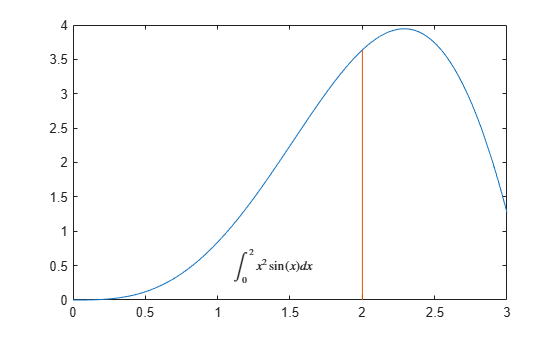## Greek Letters and Special Characters in Chart Text

You can add text to a chart that includes Greek letters and special characters using TeX markup. You also can use TeX markup to add superscripts, subscripts, and modify the text type and color. By default, MATLAB® supports a subset of TeX markup. To use additional special characters, such as integral and summation symbols, you can use LaTeX markup instead. This example shows how to insert Greek letters, superscripts, and annotations into chart text and explains other available TeX options.

### Include Greek Letters

Create a simple line plot and add a title. Include the Greek letter $\pi$ in the title by using the TeX markup \pi.

x = linspace(0,2*pi); y = sin(x); plot(x,y) title('x ranges from 0 to 2\pi')### Include Superscripts and Annotations

Create a line plot and add a title and axis labels to the chart. Display a superscript in the title using the ^ character. The ^ character modifies the character immediately following it. Include multiple characters in the superscript by enclosing them in curly braces {}. Include the Greek letters $\alpha$ and $\mu$ in the text using the TeX markups \alpha and \mu, respectively.

t = 1:900; y = 0.25*exp(-0.005*t); figure plot(t,y) title('Ae^{\alphat} for A = 0.25 and \alpha = -0.0005') xlabel('Time') ylabel('Amplitude')Add text at the data point where t = 300. Use the TeX markup \bullet to add a marker to the specified point and use \leftarrow to include an arrow pointing to the left. By default, the specified data point is to the left of the text.

txt = '\bullet \leftarrow 0.25t e^{-0.005t} at t = 300'; text(t(300),y(300),txt)### TeX Markup Options

MATLAB supports a subset of TeX markup. Use TeX markup to add superscripts and subscripts, modify the text type and color, and include special characters. MATLAB interprets the TeX markup as long as the Interpreter property of the text object is set to 'tex' (the default).

Modifiers remain in effect until the end of the text. Superscripts and subscripts are an exception because they modify only the next character or the characters within the curly braces. When you set the Interpreter property to 'tex', the supported modifiers are as follows.

ModifierDescriptionExample
^{ }Superscript'text^{superscript}'
_{ }Subscript'text_{subscript}'
\bfBold font'\bf text'
\itItalic font'\it text'
\slOblique font (usually the same as italic font)'\sl text'
\rmNormal font'\rm text'
\fontname{specifier}Font name — Replace specifier with the name of a font family. You can use this in combination with other modifiers.'\fontname{Courier} text'
\fontsize{specifier}Font size —Replace specifier with a numeric scalar value in point units.'\fontsize{15} text'
\color{specifier}Font color — Replace specifier with one of these colors: red, green, yellow, magenta, blue, black, white, gray, darkGreen, orange, or lightBlue.'\color{magenta} text'
\color[rgb]{specifier}Custom font color — Replace specifier with a three-element RGB triplet.'\color[rgb]{0,0.5,0.5} text'

This table lists the supported special characters with the Interpreter property set to 'tex'.

Character SequenceSymbolCharacter SequenceSymbolCharacter SequenceSymbol

\alpha

α

\upsilon

υ

\sim

~

\angle

\phi\leq

\ast

*

\chi

χ

\infty

\beta

β

\psi

ψ

\clubsuit

\gamma

γ

\omega

ω

\diamondsuit

\delta

δ

\Gamma

Γ

\heartsuit

\epsilon

ϵ

\Delta

Δ

\spadesuit

\zeta

ζ

\Theta

Θ

\leftrightarrow

\eta

η

\Lambda

Λ

\leftarrow

\theta

θ

\Xi

Ξ

\Leftarrow

\vartheta

ϑ

\Pi

Π

\uparrow

\iota

ι

\Sigma

Σ

\rightarrow

\kappa

κ

\Upsilon

ϒ

\Rightarrow

\lambda

λ

\Phi

Φ

\downarrow

\mu

µ

\Psi

Ψ

\circ

º

\nu

ν

\Omega

Ω

\pm

±

\xi

ξ

\forall

\geq

\pi

π

\exists

\propto

\rho

ρ

\ni

\partial

\sigma

σ

\cong

\bullet

\varsigma

ς

\approx

\div

÷

\tau

τ

\Re

\neq

\equiv

\oplus

\aleph

\Im

\cup

\wp

\otimes

\subseteq

\oslash

\cap

\in

\supseteq

\supset

\lceil

\subset

\int

\cdot

·

\o

ο

\rfloor

\neg

¬

\nabla

\lfloor

\times

x

\ldots

...

\perp

\surd

\prime

´

\wedge

\varpi

ϖ

\0

\rceil

\rangle

\mid

|

\vee

\langle

\copyright

### Text with Mathematical Expression Using LaTeX

By default, MATLAB interprets text using TeX markup. However, for more formatting options, you can use LaTeX markup instead. For example, you can include mathematical expressions in text using LaTeX. To use LaTeX markup, set the Interpreter property for the Text object to 'latex'.

For this example, plot $y={x}^{2}\mathrm{sin}\left(x\right)$ and draw a vertical line at $x=2$. Add text to the graph that contains an integral expression using LaTeX markup.

x = linspace(0,3); y = x.^2.*sin(x); plot(x,y) line([2,2],[0,2^2*sin(2)]) str = '$$\int_{0}^{2} x^2\sin(x) dx$$'; text(1.1,0.5,str,'Interpreter','latex')